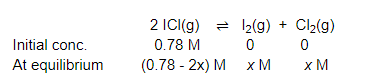# What is the equilibrium concentration of each of the substances in the equilibrium when the initial concentration of ICl was 0.78 M?

Question:

What is the equilibrium concentration of each of the substances in the equilibrium when the initial concentration of ICl was 0.78 M?

$2 \mathrm{ICl}(\mathrm{g}) \rightleftharpoons \mathrm{I}_{2}(\mathrm{~g})+\mathrm{Cl}_{2}(\mathrm{~g}) ; \mathrm{K}_{\mathrm{C}}=0.14$

Solution:

The given reaction is:Now, we can write, $\frac{\left[\mathrm{I}_{2}\right]\left[\mathrm{Cl}_{2}\right]}{[\mathrm{ICl}]^{2}}=K_{\mathrm{C}}$

$\Rightarrow \frac{x \times x}{(0.78-2 x)^{2}}=0.14$

$\Rightarrow \frac{x^{2}}{(0.78-2 x)^{2}}=0.14$

$\Rightarrow \frac{x}{0.78-2 x}=0.374$

$\Rightarrow x=0.292-0.748 x$

$\Rightarrow 1.748 x=0.292$

$\Rightarrow x=0.167$

Hence, at equilibrium,

$[\mathrm{ICl}]=\left[\mathrm{I}_{2}\right]=0.167 \mathrm{M}$

$[\mathrm{ICl}]=(0.78-2 \times 0.167) \mathrm{M}$

$=0.446 \mathrm{M}$